# Rotating Grids in Squares and Hexagons

## Are you folding from crease patterns and looking to level up your tessellations?

You don’t have a laser cutter or Cricut scorer, but you still want to make stunning tessellations without guessing and iterating over and over. You’ve seen some tessellations that are mysteriously well-aligned with the edge of the paper, but don’t know how it’s done.

Sounds familiar, right?

The good news is that there’s a fairly simple solution - rotated grids! These grids take into account the alignment of the repeating elements of the tessellation with the grid, and then allow you to align the edges of the paper with those repeating elements, at the location of your choosing.

You may have seen rotated grids before in tutorials for the Triangle Twist tessellation or variations of Fujimoto’s Clover where the grid is perpendicular to the normal edge-aligned grid. Mark Leonard also used a 2:1 grid alignment for the Waterbomb tessellation back in 2004. I learned about arbitrary rotated grids from Elseh (@zamanulli on IG), and just like when I learned the odd gridding reference method, it was too good to not share.

## What exactly does that look like?

Here’s some examples of a tessellation that was made with and without rotating the grid.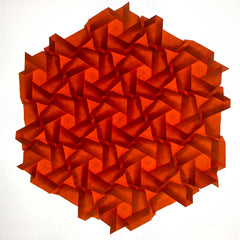Dense Compound Hexagons, without and with grid rotation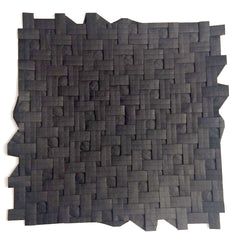Open Alternating Weave, without and with grid rotation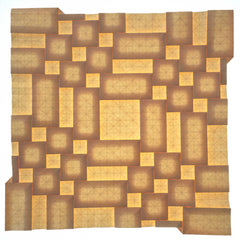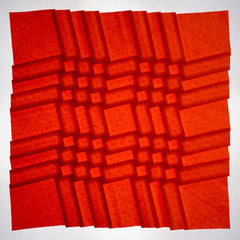Gapped Clusters, without and with grid rotation

You can see that the rotated tessellations have the same repeating elements as the normal ones - they're just aligned with the edges.

This completely eliminates any awkward wedges of paper at the edges of the design and leaves a polished, intentional-looking finish.

## I’m convinced - how does this work?Mixed Compound Squares Tessellation, my designLens Stars, independently discovered by many origamists, including me

1. Look at your crease pattern. Find your center point, and draw a line to the same point in another pattern repetition. There are many options for the second point - the closer the point, the easier the reference (generally), and among equivalent options I always choose the one closest to horizontal to the right of my center point.

• If your line is exactly diagonal to the grid ( $// $ ) like in the hexagon example above, you can skip the reference calculations and fold opposite corners together instead.
2. Count the $// $ and $// $ grid units from the first point to the second point, and plug them into the appropriate formula for the square grid reference or triangle grid reference.

• Note: $// $ values on the square grid are positive for positive slopes and negative for negative slopes.
3. Count from your center point along a grid line until you find a good place for the edge of the paper. As with normal gridding methods, you’ll want to prioritize powers of two for simplicity or at least find a multiple of two or four.

• This count will be the number of divisions between the center and the edge, which is half of the total divisions.
• The physical size of the divisions will be slightly larger than normal on a square, and slightly smaller on a hexagon.
4. Mark the reference you calculated in step 2 on the edges of your paper. The triangle grid reference is only for hexagonal paper - see the last section for referencing triangle grids on a square.

• Make the mark going up the right-most edge from the bottom right corner.
• Having a second piece of paper to use as a template for the references really cuts down on marks on the paper. I have one each for thirds, fifths, and sevenths.
• Think hard about which side of the paper you want your harshest marks from the grid lines before you set your references. This is a personal preference, but I prefer my harshest grid marks on the front where everyone can see them, but I prefer the harsh marks from the references on the back, so I mark the references as viewed from the front and flip the paper over to fold the grid.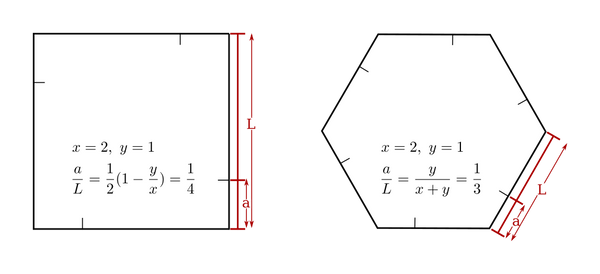5. Make valley folds between the reference marks on opposite edges. These creases will intersect in the exact center of the paper.

• Square grids bring opposite reference marks together, making a crease between the other pair of references.
• Triangle grids bring two “bottom” references to meet two “top” references, making a crease between the left and right references.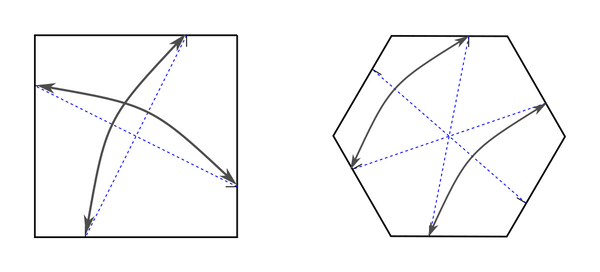6. Fold the references in to the center. This is why you need at least a multiple of two for your grid count from the center to the edge.

• Square grid references go exactly to the center intersection. You can also start adding diagonals for a 45-degree grid at this point, although I advise caution to avoid confusing the diagonals with the main grid.
• Triangle grid references go in adjacent pairs to the central line. You can also fold the outer creases that end at the reference points once the inner creases have been folded now that more references are available for those lines.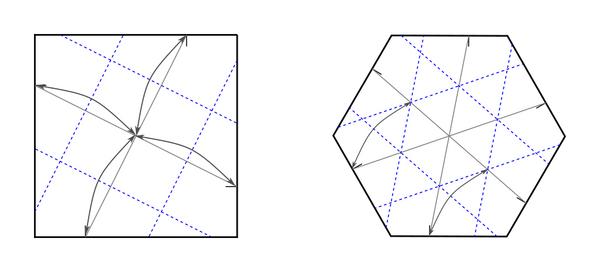7. Fold odd grid divisions as needed, and continue dividing by two until you reach your target grid divisions.

8. Precrease as needed, and collapse your tessellation as usual.

9. Marvel in the majesty of what you have created and share the good news with another folder.

Here’s what the Lens Stars example from the top of the section looks like on a 32-fold rotated (diagonal) grid:and here's two possibilities for rotating the Mixed Squares example, with the one given in the diagram pictured first: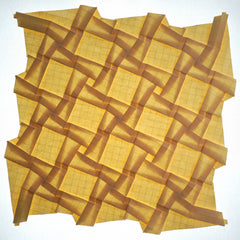## Where did those equations come from?

### Square Grid Proof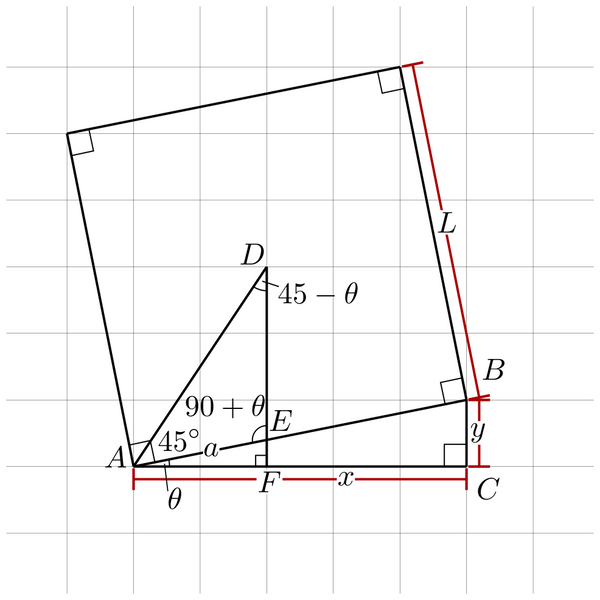Given the above diagram, with only $// $ and $// $ known, what is $// $ ? Point $// $ is the center of the square, $// $ is perpendicular to $// $ , and $// $ is the angle bisector of the corner of the square at $// $ .

Since $// $ is parallel to $// $ (both are $// $ to $// $ ), $// $ since all three angles are the same.

Since $// $ and $// $ are supplementary, $// $ since the sum of angles in a triangle is $// $ , and $// $ . This leaves $// $ with $// $ degrees since $// $ .

We know that $// $ and $// $ from $// $ .

By the Law of Sines,

and so

which gives us precisely equation $// $ !

This equation covers the range $// $ (aligned on lower diagonal, $// $ ) to $// $ (aligned on upper diagonal, $// $ ).

### Triangle Grid Proof (on a hexagon)Given the above diagram, with only $// $ and $// $ known, what is $// $ ? Point $// $ is the center of the hexagon, and $// $ is the bisector of $// $ .

$// $ since the interior angles of $// $ sum to $// $ and $// $ .

Therefore, $// $ also since it is opposite $// $ .

$// $ since $// $ represents one of the six equilateral triangles making up a hexagon, so $// $ .

By the Law of Sines,

for $// $ , and so

and also

By the Law of Sines for $// $ ,

and so

which gives us precisely equation $// $ !

This equation covers the range from $// $ (aligned on lower grid line, $// $ ) to $// $ (aligned on upper grid line, $// $ ) and there is no sign flipping to take into account.

## Bonus: Triangle grids on squares

Full disclosure: You’ll need a calculator and Reference Finder to fold these references. It’s not simple fractions like the triangle grid on a hexagon. Also, I’m confident in the math, but I haven’t tested it in practice yet.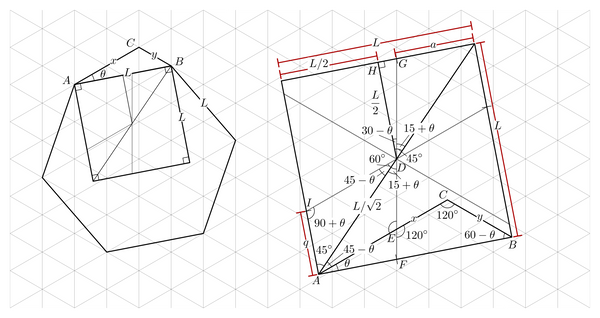I’ve shifted $// $ down to the bottom edge of the square here so we can take advantage of some convenient intersections. Point $// $ is the center of the square, and $// $ is a perpendicular bisector of the top edge. $// $ bisects the bottom left corner of the square, points $// $ and $// $ are rotationally equivalent, and $// $ lies on triangular grid lines and measures $// $ .

This proof starts exactly like the triangle grid on hexagons, with the Law of Sines for $// $ .

From there, we calculate $// $ and $// $ , so we can plug them back in later.

Next, we’ll calculate all the relevant angles.

$// $ is defined as $// $ , and since $// $ bisects $// $ that leaves $// $ with $// $ degrees. $// $ and $// $ are aligned with the triangular grid, so their intersection is at $// $ and $// $ . That gives $// $ and $// $ . Since $// $ is defined at $// $ , that makes $// $ . $// $ is opposite the angle with endpoints defining $// $ , and since the diagonal and $// $ make a $// $ angle, that leaves $// $ . Last but not least, $// $ . That gets us caught up with the diagram, and we can continue with the proof!

We’ll start with finding $// $ , then continue to find $// $ .

So, there’s our reference for the previously-vertical axis as it intersects with the top and bottom edges of the square. Notice that $// $ for $// $ .

Let’s turn towards the lower left/upper right axis.

By the Law of Sines on $// $ ,

This gives us

which is the reference for the second axis for the triangle grid. Note that $// $ when $// $ , which is exactly what you would expect with the standard vertical orientation of the grid on the square.

With those four references (two for $// $ and two for $// $ ), we can fold the third axis by folding the first two axes together.

The references at $// $ and $// $ are not aligned on the triangle grid (with one exception), so you’ll need to decide which pair of references to fold in to the center line.

## Conclusion

I’d love to see what y’all fold with rotated grids - tag me (@gatheringfolds) on IG or FB and show me what you’ve got!

Rotating the grids was a big step up for me, and it may take some trial and error to get used to it in your folding. I’m planning on releasing several video tutorials, so if it still seems mysterious don’t worry - videos are coming soon!

Good luck on all of your folding adventures, and happy folding, everyone!

Gathering Folds